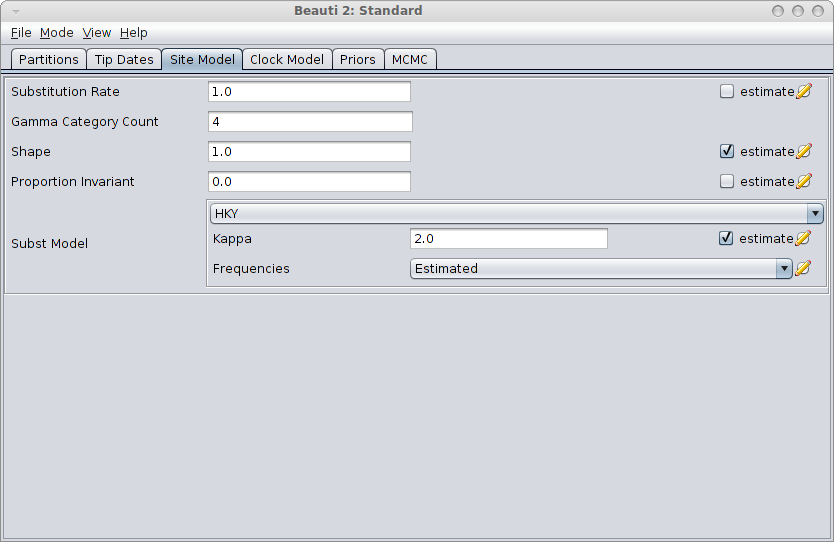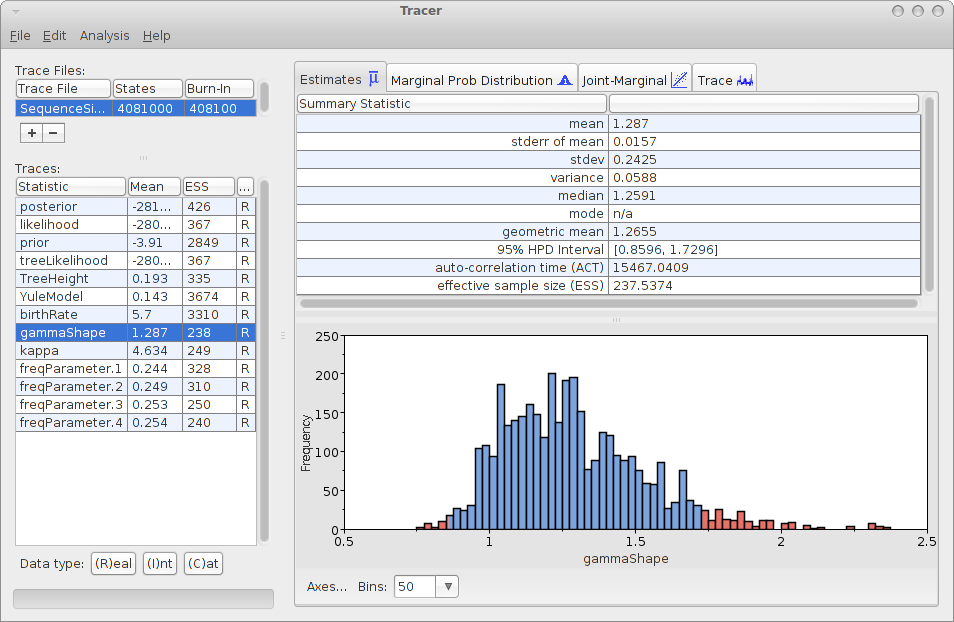# Simulation studies with BEAST 2

28 April 2014 by Remco Bouckaert

Ever wondered whether the gamma shape that is estimated has any relation to the gamma shape that generated the data? Probably not, but there are many cases where you want to find out whether a model that you use has any relation to the process that generated the data. If so, you probably want to do a simulation study and observe how parameters that generate sequence data have an impact on the parameters of the model that you use to analyse the data.

BEAST has a number of tools that you can use to do a simulation study. Here, we will check what will happen when you change the rate heterogeneity and how it impacts the gamma shape estimate when using the gamma rate heterogeneity model.

## Generating an alignment

First of all, we will set up a simulator using the SequenceSimulator class in BEAST. At the time of writing, no GUI is available, so some XML hacking is required.

We will generate a sequence from a 3 taxa fixed tree using the HKY substitution model (kappa=5) and 4 rate categories with gamma shape ranging from 0.2 to 15. The following XML shows how to do this:

If you save the XML as simluator.xml you can run it using BEAST, and it will produce a file called gammaShapeSequence.xml containing a sequence alignment with 10000 characters.

## Setting up an analysis

Next step is to create a BEAST MCMC analysis file. The easiest way to do this is using BEAUti. Open BEAUti using the (default) Standard template. Import the alignment from the generated file gammaShapeSequence.xml. Go to the Sitemodel panel, and change the substitution model to HKY. Then, set the number of categories to 4, and click the estimate checkbox next to the shape. The panel should look something like this:Save the file as analysis.xml. After running the analysis with BEAST, and using tracer to inspect the log file SequenceSimulator.log, tracer could report something like this:As you can see, the gamma shape is estimated as 1.287 with a 95% HPD Interval of [0.8596, 1.7296], so the original gamma shape value of 1.0 is in the 95% HPD Interval. So, it appears that the gamma shape parameter can be estimated. How about the bias? Perhaps we should run the simulation a few more time and see how it behaves over say 10 independently generated alignments.

## Repeating this 10x

To repeat the above — simulate sequences, merge with an analysis, and run the analysis — say 10 times, we can adjust the XML script simply by adding the following:

• Add an iterations attribute to the run element, setting iterations="10".
• Add a merge element indicating that the generated alignment should be merged with the XML representing an analysis — it replaces the alignment in a BEAST XML file with the one generated by the SequenceSimulator. Since we just created the file analysis.xml with the kind of analysis we want to run, we can use that file.
• Note that you can specify more than one merge file, and every template file has its alignment replaced with the same generated alignment. This is handy if you want to find out how model misspecification impacts your estimates.

The XML should look something like this:

Save as simulator10.xml in the directory containing analysis.xml and when you run the XML file with BEAST, there should be 10 files generated, named analysis-0.xml, analysis-1.xml, up to analysis-9.xml. You could run the ten XML files with BEAST, but you will quickly find out that every log file has the same name — running each file in its own directory, or renaming log files after running an analysis is quite labourious.

Fortunately The sequence simulator, before it replaces the alignment in the analysis file, replaces every instance of “\$(n)” (dollar followed (n)) by the iteration number. So, if we change the file names of the loggers in the analysis.xml file like so:

From

to

And, from

to

It is probably also a good idea to set the chainLength="4000000" in analysis.xml. Rerun BEAST on simluator10.xml.

Now we can run the 10 simulator files and get log file SequenceSimulator-0.log, SequenceSimulator-1.log, … SequenceSimulator-9.log.

Analysing the log files can be done with tracer with the 10 log files. For the 10 log files, we can select the combined log, which looks something like this:The mean estimate over 10 runs is 1.0752 with 95% HPD Interval of [0.7423, 1.503], so the gamma shape used for generating the data is close to the mean and it is firmly in the 95% HPD interval.

## Repeating 10x for 21 different gamma shape values

So, it looks like the gamma shape value can be infered when the value is 1.0. How about other values? To test this, we can change the simulator.xml file for different values of the shape parameter, and run the analysis 10 times for each gamma shape.

## Analysing with LogAnalyser and R

Doing this for 21 values of the shape parameter gives 210 log files, which become a bit cumbersome to analyse with tracer. If you would have run the same analysis with a different template (e.g. with a GTR model instead of HKY), you get another 210 log files.

BEAST has a LogAnalyser that produces similar output to tracer in table format. To run it, you can use

Running it on one of the trace logs produces output like this:

For this run, the estimated gamma shape is 1.198491 with an ESS of 202.

By specifying the prefix directive, you can add a column to identify the run by a prefix value. For example, setting -Dprefix=1.0 like so:

This makes it easy to have for example an R script to find the estimates associated with a particular value used for generating the data.

If you specify more than one indicators, for example the iteration number and the shape parameter, just insert them in braces, like so

The output now has two columns, with headers labeled prefix0 prefix1 and column values 9 1.0

Using the LogAnalyser, you can create a text file containing the estimates for all logs. Doing this for various values of gamma shape,

You can create a log.dat that you can use in R for a plot. In R, we can do the following — assuming we generated alignments with shape parameter values 0.2, 0.3, 0.4, 0.5, 0.6, 0.7, 0.8, 0.9, 1.0, 1.2, 1.4, 1.6, 1.8, 2.0, 2.5, 3.0, 4.0, 5.0, 7.5,10.0, 15.0:

This gives the following graph showing the estimate of shape parameter against the value used for simulating the data. Over all, there is a close match, except for large values of the shape parameter.